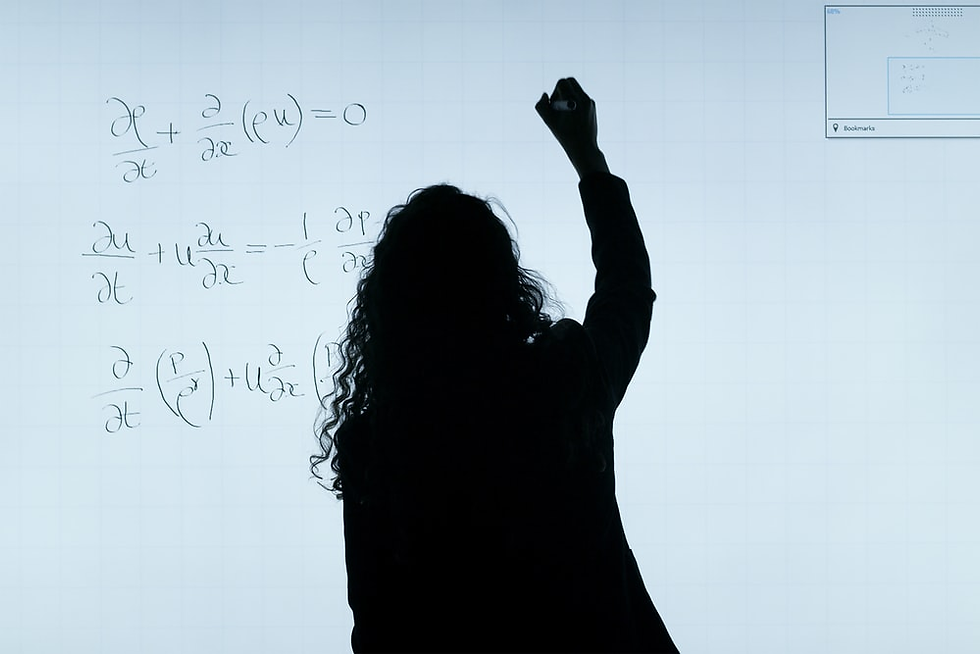top of page
Search

# How to Learn Math FormulasMany students struggle with learning math formulas. Whether you are a fifth-grader or a candidate for SAT, memorizing every math formula can be challenging. Sometimes, no matter how hard you try, knowing all the variables and coefficients of a mathematical equation may seem impossible.

### How to Learn Math Formulas

If learning math formulas is not your favorite thing, stop worrying! Here is a list of ways to learn math formulas.

If you have a math class tomorrow, it is best to go through the lesson beforehand. Going through all the new formulas ahead of time can help you to better understand them before the teacher covers them in class. Moreover, when you cover the formulas before the starting of the lesson, you will remember some parts of them, which can help you memorize them in the class later.

### Meaning

Math formulas may seem meaningless to you; however, you can associate each number and letter with a meaning of your own. Your brain will likely remember the formulas if you relate them to something you like. For example, when learning about an ellipse, you can imagine its shape while learning its formulas to help differentiate the formulas from the rest of the mathematical formulas.

### Practice

Practice makes you perfect! The more you practice, the better you will memorize the formulas. It may require some time; however, after a few days, you will be able to remember each formula if you consistently practice the numerical problems at home.

If you face any difficulty in solving mathematical questions, you can enroll yourself at Pinnacle Xplore to learn math. Then, with the help of our private tutors, you will understand the mathematical concepts and formulas without a single worry.